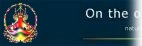Table of contents Overview A sample run The AI matrix List of parameters Further references Credits Bug report   List of parameters Main matrix rows: the number of rows of the main matrix. Main matrix columns: the number of columns of the main matrix. Element matrix rows: the number of rows of an element matrix, ie. a main matrix node. Element matrix columns: the number of columns of an element matrix, ie. a main matrix node. Block factor: how many times a node is processed by the algorithm before moving on to the next node. Connection depth: the level of the virtual tree (ie., a traversal tree without the usual explicitly declared structure). Connectivity: expressed in percentage terms, the number of nodes each node of the main matrix is connected to. High end of element number range: the maximum integer used in element matrix nodes. Number of input regions: how many regions (of equal size, except for the remainder) the number of input nodes are divided into. Number of output regions: how many regions (of equal size, except for the remainder) the number of output nodes are divided into. Active input region: which of the available input regions is selected to provide input to the inner matrix. Active output region: which of the available output regions is selected to collect the output from the inner matrix.